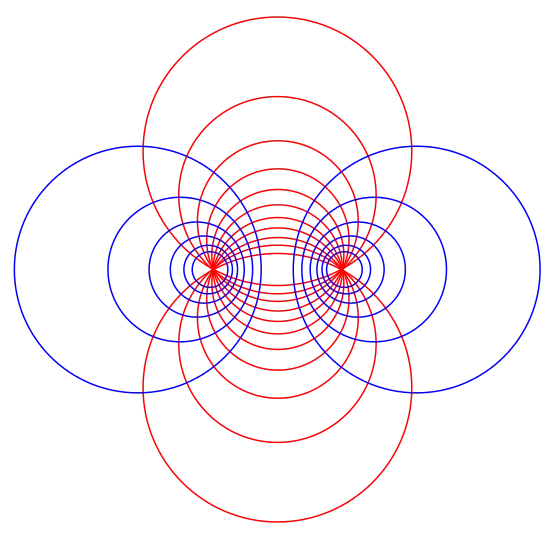# Amarnath

## CBSE Term 1 Sample Papers MCQ 2021-22

here, one can find the CBSE term 1 Sample Papers , sample mcq quizzes of cbse new pattern of term 1 of 2021-2022, for all the classes and all the subjects , Class X CBSE term 1 Sample Papers Sanskrit…

## Differential Equations Class 12 notes | IIT-JEE study materialSo, in calculus we are starting the topic of differential equations, we will be seeing what does a differential equation exactly means, what are partial differential equations, the significance of differential equations in various disciplines is very vast, we will…

## Applications of Derivatives class 12 revision notes | IIT-JEE notes, study materialWe have analyzed the concept of derivative/Differentiation of a function, this concept is widely used in various fields like math, physics, engineering sciences, etc, derivatives are used to analyze any function by calculating its first and second derivative, Hence we…

## Methods of Differentiation | Concept Booster IIT-JEE Class 12 notesMethods of Differentiation Here we will learn methods of Differentiation, We had already studied the two meanings of derivative(differentiation) of a function which is the geometrical and physical meanings. Since we know what does the differentiation of a function means…

## Differentiability Class 12 | IIT JEE Notes, Concept BoosterDifferentiability The concept of the differentiability (derivability) of a function tells us about whether a given function will be differentiable or not. If the function is differentiable then we will use Methods of differentiation to differentiate a function. Actually, this…